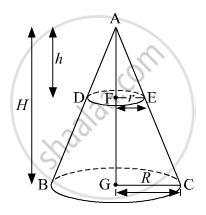Share

# The Height of a Cone is 30 Cm. from Its Topside a Small Cone is Cut by a Plane Parallel to Its Base. If Volume of Smaller Cone is 1/27 of the Given Cone, Then at What Height It is Cut from Its Base? - CBSE Class 10 - Mathematics

#### Question

The height of a cone is 30 cm. From its topside a small cone is cut by a plane parallel to its base. If volume of smaller cone is 1/27 of the given cone, then at what height it is cut from its base?

#### Solution

Let the radius and height of the bigger cone be R and H, respectively.

Given: H = 30 cm

Let the radius and height of the smaller cone be r and h, respectively.Now, in ∆AFE and ∆AGC,

∠AEF = ∠ACG (Corresponding angles)

∠AFE = ∠AGC (90° each)

∴ ∆AFE~∆AGC (AA similarity)

=> (AF)/(AG) = (FE)/(GC)

=> h/H = r/R  ....(1)

it is given that

Volume of the smaller cone = 1/27 xx "Volume of the bigger cone"

=> 1/3 pir^2h = 1/27 xx 1/3 piR^2H

=>(r/R)^2 xx h/H = 1/27

=> (h/H)^2 xx h/H = 1/27        [Using (1)]

=> (h/H)^3 = 1/27

=> h/H = 1/3

:. h = 1/3 xx H = 1/3 xx 30 = 10 cm

Now

FG = AG − AF = 30 cm − 10 cm = 20 cm

Thus, the small cone is cut at the height of 20 cm from the base.

Is there an error in this question or solution?

#### Video TutorialsVIEW ALL 

Solution The Height of a Cone is 30 Cm. from Its Topside a Small Cone is Cut by a Plane Parallel to Its Base. If Volume of Smaller Cone is 1/27 of the Given Cone, Then at What Height It is Cut from Its Base? Concept: Frustum of a Cone.
S